Courses

# Test: Power Electronics - 3

## 10 Questions MCQ Test GATE Electrical Engineering (EE) 2022 Mock Test Series | Test: Power Electronics - 3

Description
This mock test of Test: Power Electronics - 3 for Electrical Engineering (EE) helps you for every Electrical Engineering (EE) entrance exam. This contains 10 Multiple Choice Questions for Electrical Engineering (EE) Test: Power Electronics - 3 (mcq) to study with solutions a complete question bank. The solved questions answers in this Test: Power Electronics - 3 quiz give you a good mix of easy questions and tough questions. Electrical Engineering (EE) students definitely take this Test: Power Electronics - 3 exercise for a better result in the exam. You can find other Test: Power Electronics - 3 extra questions, long questions & short questions for Electrical Engineering (EE) on EduRev as well by searching above.
QUESTION: 1

### In a type A chopper, the maximum value of Ripple current is

Solution:

Concept:

Steady state ripple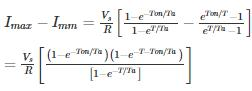The peak to peak ripple current has maximum value when duty cycle ∝ = 0.5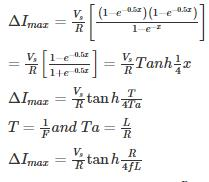In case 4FL ≫ R, then tan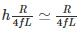Calculation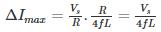∴ maximum value of Ripple current is inversely proportional to chopping frequency and the circuit Inductance.

*Answer can only contain numeric values
QUESTION: 2

### A boost – regulator has an Input voltage 8V and the average output voltage of 24 V the duty cycle is _

Solution:

We known Boost regulator is a step – up chopper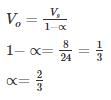*Answer can only contain numeric values
QUESTION: 3

### A DC chopper operates on 220 V dc and frequency of 500 Hz, feeds on RL load of the output voltage of chopper is 180 V the ON time of the chopper is –(in msec)

Solution:

Vdc = 220V, f = 500 Hz, Vo = 180 V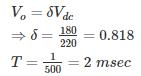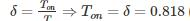(2×10−3) = 1.636 msec

QUESTION: 4

DC chopper feed an RLE load. If the value of E increased by 20%, the current ripple

Solution:

Current ripple does not depend on the value of E
ΔI= Vdc ∝ (1−∝)T

QUESTION: 5

A class C chopper is operated from 230 V battery the load is a dc motor with R = 0.15 Ω, L = 12 mH and Eb = 120 V. The duty cycle of the chopper to achieve regenerative braking at the rated current of 12 ampere would be equal to

Solution:

First find rated load current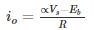For Regeneration braking load current should be negative.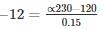-1.8 = ∝230 – 120

230∝ = 118.2

∝ = 0.5139

∴ Duty cycle of chopper = 51.39 %

*Answer can only contain numeric values
QUESTION: 6

A single - quadrant chopper is operating with the following specification: Ideal battery of 240 V; on time (Ton) = 1.5 ms, OFF time = 2 ms. The Form factor factor will be –

Solution:

Concept:

For Single – quadrant (type A) Chopper

Average value Vo = ∝ Vs
R.M.S value Vrms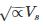where ∝ = Ton/T
We known the form factor F.F =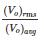Calculation:

Form factor =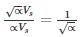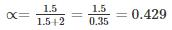∴ form factor = 1.528

*Answer can only contain numeric values
QUESTION: 7

A class C chopper is operated from a 220 V battery. The load is a dc motor with R = 0.1 Ω, L = 10 mH and E= 100 V. The rated current is 10 A. The duty cycle in motoring mode is

Solution: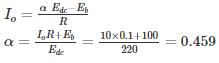QUESTION: 8

A buck converter is to be designed where Vs = 10V, Vo = 5V,R = 500Ω. The switching frequency is decided to be 20 kHz. Allowable current ripple is 5 mA. The optimum value of inductor is

Solution: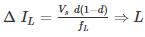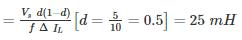*Answer can only contain numeric values
QUESTION: 9

A step-up chopper shown in figure is to deliver 3A
In to the 10 Ω load. The battery voltage is 12 V, L = 20 μH, C = 100 μF and chopper frequency is 50 kHz. Determine the battery current variation in ampere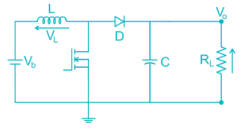Solution:

Calculation:

Vo = IoRL = 3 × 10 = 30 V

Vb = Vo (1 – D)
1−D = 12/30 = 0.4
D = 0.6

Periodic time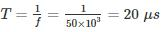On time = DT = 0.6 × 20 × 10-6 = 12 μs

over an on-time the change in battery current will be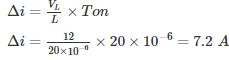Concept: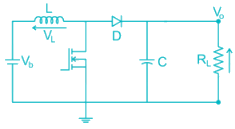When the transistor is switched on, current ramps up in the inductor and energy is stored.

When the transistor is switched off, the inductor voltage reverses and acts together with the battery voltage to forward bias the diode, transferring energy to the capacitor.

When the transistor is switched on again, load current is maintained by the capacitor, energy is stored in the inductor and the cycle can start again.

The value of the load voltage is increased by increasing the duty cycle or the on-time of the transistor.

During the transistor on-period, assuming an ideal inductor and transistor

Vb = VL = Ldi/dt

Vb/s = (sL) i(s)

i(s) = Vb/s2L

i(t) = (Vb/L)t = kt

Over an on-time of TON, the change of battery current will be

Δi = k TON

During the off-period

V= Vb + V= Vb + L(di/dt) = Vb + L(Δi/Δt)

= Vb + L(Vb/L)TON /Toff = Vb(1 + TON /Toff)

Let the duty cycle

D = TON T, and Toff = T – TON = T(1 - D). Now

Vo = Vb (1 – DT/T(1 – D))

which simplifies to

Vo = Vb/(1 - D)

In an ideal circuit, VbI= V0I0. Therefore, from eq.

Ib = (Vo/Vb)Ib = Io/(1 – D)

Ib = Ib + Δi/2

Ibo = Ib – Δi/2

When the steady variation of battery current has been reached, the variation will be between lo and I1, as shown in.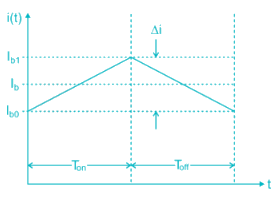QUESTION: 10

In the circuit shown below, if load R = 500 Ω, switching frequency is 25 kHz and peak to peak ripple current of inductor is limited to 0.9 A then the filter inductance L is"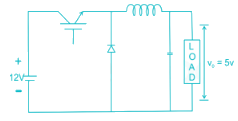Solution:

For Buck Converter
V= αVdc ⇒ α = 5/12
The peak to peak ripple current is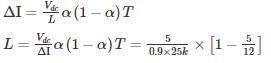L = 129.62 μH